Important Update
The Guide Feature will be discontinued after December 15th, 2023. Until then, you can continue to access and refer to the existing guides.Gaurav Singhal

# Artistic Neural Style Transfer with PyTorch

• Jun 9, 2020
• 4,764 Views
• Jun 9, 2020
• 4,764 Views
Data
Data Analytics
Machine Learning
PyTorch

## Overview

In this guide, you will implement the algorithm on Neural Network for Artistic Style Transfer (NST) in PyTorch. This algorithm will allow you to get a Picasso-style image. It does so by creating a new image that mixes the style (painting) of one image and the content (input image) of the other.

Thanks to Leon A. Gatys, et al. for their contribution in their paper, A Neural Algorithm of Artistic Style.

To make the model faster and more accurate, a pre-trained VGG-Net-19 (Visual Geometry Group) is used. The model is trained on ImageNet images and can be downloaded from Pytorch API.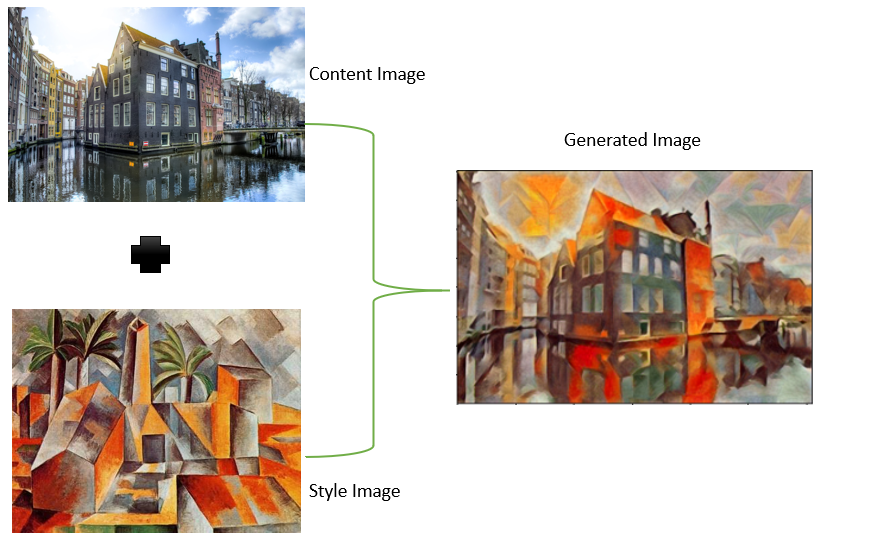The theory behind artistic neural transfer has been covered in previous guides that were based on the TensorFlow framework. It is highly recommended that you go through these guides first. Part 1 talks about theoretical aspects and VGG-Net, and Part 2 talks about losses involved in creating AI digital art.

The guide will be a code walkthrough of the PyTorch implementation. Below is an outline of the process.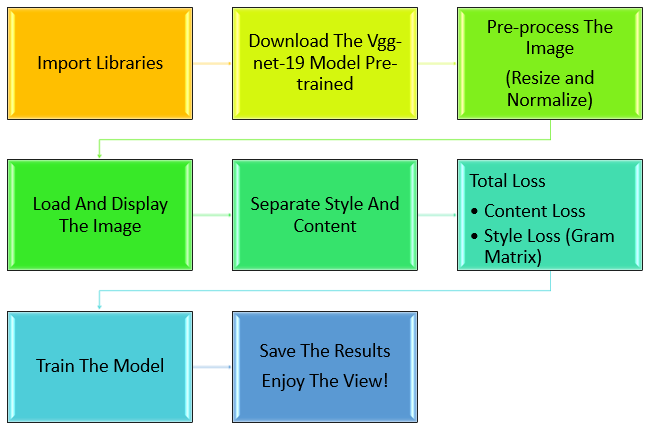## Importing Libraries

To work with PyTorch, import the `torch` library. The `PIL` image library will manipulate the image. From the function `torchvision`, you will import `model` class and call for `vgg19` model.

``````1%matplotlib inline
2
3from PIL import Image
4from io import BytesIO
5import matplotlib.pyplot as plt
6import numpy as np
7
8import torch
9import torch.optim as optim
10import requests
11from torchvision import transforms, models``````
python

## Build the Model

Load the VGG-Net-19 model and keep `pretrained=True`. The number 19 denotes the number of layers involved in the network. VGG has its use in the classification problem (face detection) as well. But in NST, you are only dealing with features. The `param.requires_grad_()` will freeze all VGG parameters since you're only optimizing the target image.

``````1vgg = models.vgg19(pretrained=True).features
2
3for param in vgg.parameters():
python

But there's a catch in PyTorch! Here you have to check if GPU is available in your system. If it is, then move the `vgg` model to GPU. If it's not, then the model will run on CPU. And if you don't have a GPU? No worries--you can use Google Colab.

``````1device = torch.device("cuda" if torch.cuda.is_available() else "cpu")
2
3vgg.to(device)``````
python

The smaller the image size, the faster the processing speed will be. The transform class of `torchvision` plays an important role while pre-processing and normalizing the image.

``````1def load_image(img_path, max_size=400, shape=None):
2
3    if max(image.size) > max_size:
4        size = max_size
5    else:
6        size = max(image.size)
7
8    if shape is not None:
9        size = shape
10
11    in_transform = transforms.Compose([
12                        transforms.Resize(size),
13                        transforms.ToTensor(),
14                        transforms.Normalize((0.485, 0.456, 0.406),
15                                             (0.229, 0.224, 0.225))])
16    image = in_transform(image)[:3,:,:].unsqueeze(0)
17
18    return image``````
python
``````1style = load_image("Picasso.jpg").to(device)
2
python

Display the tensor as an image.

``````1def im_convert(tensor):
2    image = tensor.to("cpu").clone().detach()
3    image = image.numpy().squeeze()
4    image = image.transpose(1,2,0)
5    image = image * np.array((0.229, 0.224, 0.225)) + np.array((0.485, 0.456, 0.406))
6    image = image.clip(0, 1)
7
8    return image``````
python

Display the images side-by-side.

``````1fig, (ax1, ax2) = plt.subplots(1, 2, figsize=(20, 10))
2ax1.imshow(im_convert(content))
3ax1.set_title("Content-Image",fontsize = 20)
4ax2.imshow(im_convert(style))
5ax2.set_title("Style-Image", fontsize = 20)
6plt.show()``````
python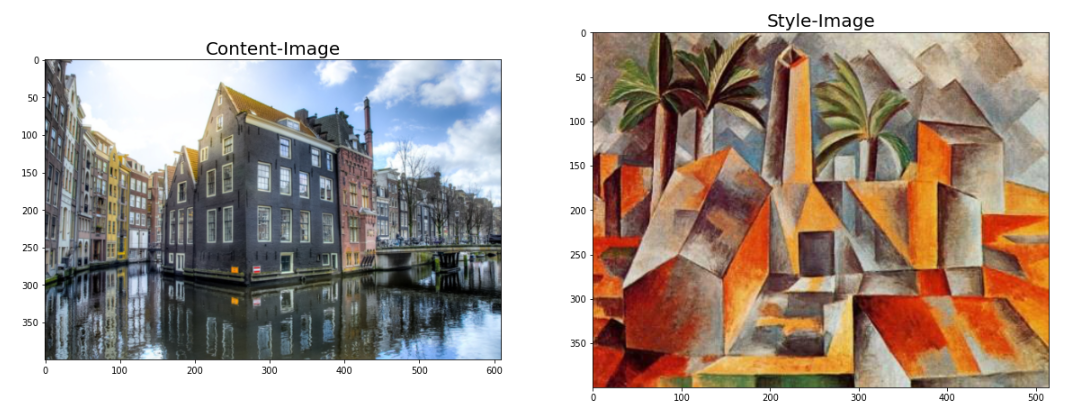``1print(vgg)``
pythons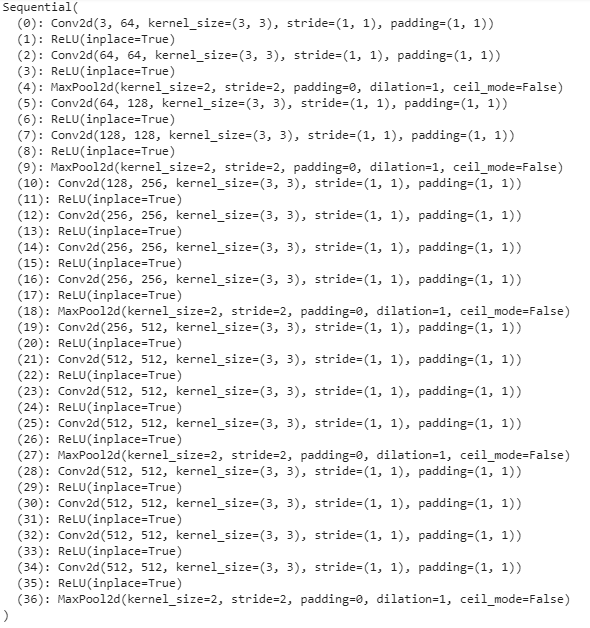## Intermediate Layers for Style and Content

Deeper layers of VGG-19 will extract the best and most complex features. Hence, `conv4_2` is assigned to extract content components. From each block, the first convolution layers (shallow layers) i.e. from `conv1_1` to `conv5_1` detects multiple features like lines or edges. Refer to the image below.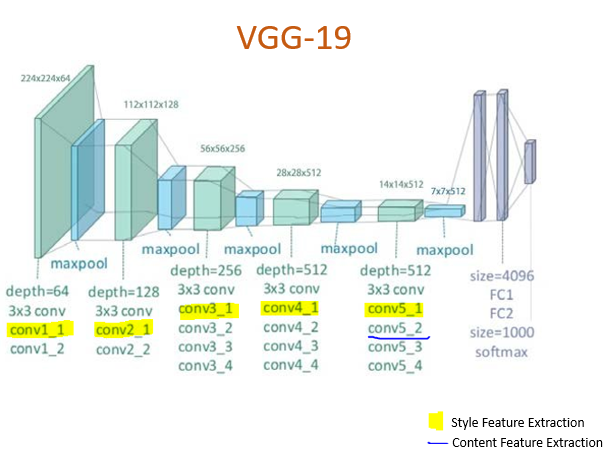``````1def get_features(image, model, layers=None):
2
3    if layers is None:
4        layers = {'0': 'conv1_1',
5                  '5': 'conv2_1',
6                  '10': 'conv3_1',
7                  '19': 'conv4_1',
8                  '30': 'conv5_2', #content
9                  '28': 'conv5_1'}
10
11    features = {}
12    x = image
13    for name, layer in model._modules.items():
14        x = layer(x)
15        if name in layers:
16            features[layers[name]] = x
17
18    return features``````
python

## Loss Functions

Calculate the gram matrices for each layer. You will need the houses and the lake in the target image. Start by cloning the content image and then iteratively change its style.

``````1def gram_matrix(tensor):
2
3    _, d, h, w = tensor.size()
4
5    tensor = tensor.view(d, h * w)
6
7    gram = torch.mm(tensor, tensor.t())
8
9    return gram ``````
python
``````1content_features = get_features(content, vgg)
2style_features = get_features(style, vgg)
3
4style_grams = {layer: gram_matrix(style_features[layer]) for layer in style_features}
5
python

## Assigning Weights

Weights are assigned on each style layer. Weight the earlier layers with a higher number to get the larger style artifacts.

``````1style_weights = {'conv1_1': 1.5,
2                 'conv2_1': 0.80,
3                 'conv3_1': 0.25,
4                 'conv4_1': 0.25,
5                 'conv5_1': 0.25}
6
7content_weight = 1e-2
8style_weight = 1e9  ``````
python

## Run the Model

These weights are used in the optimizer (Adam) to reduce the loss of the model. Define steps to update the image. Putting everything together: call the features from the VGG-Net and calculate the content loss. Get the style representation to calculate the style loss. It will weight the layer appropriately before adding it to other layers. Finally, calculate the total loss!

In a gradient, descent for NN weights are adjusted, but in NST, they are kept fixed. Instead, image pixels are adjusted. The gradients concerning the distance measure will be backpropagated to the inputs, thus transforming the inputs.

``````1show = 400
2
4steps = 7000
5
6for ii in range(1, steps+1):
7
8    target_features = get_features(target, vgg)
9
10    content_loss = torch.mean((target_features['conv4_2'] - content_features['conv4_2'])**2)
11
12    style_loss = 0
13    for layer in style_weights:
14        target_feature = target_features[layer]
15        target_gram = gram_matrix(target_feature)
16        _, d, h, w = target_feature.shape
17        style_gram = style_grams[layer]
18        layer_style_loss = style_weights[layer] * torch.mean((target_gram - style_gram)**2)
19        style_loss += layer_style_loss / (d * h * w)
20
21    total_loss = content_weight * content_loss + style_weight * style_loss
22
24    total_loss.backward()
25    optimizer.step()
26
27    if  i % show == 0:
28        print('Total loss: ', total_loss.item())
29           plt.imshow(im_convert(target))
30        plt.show()``````
python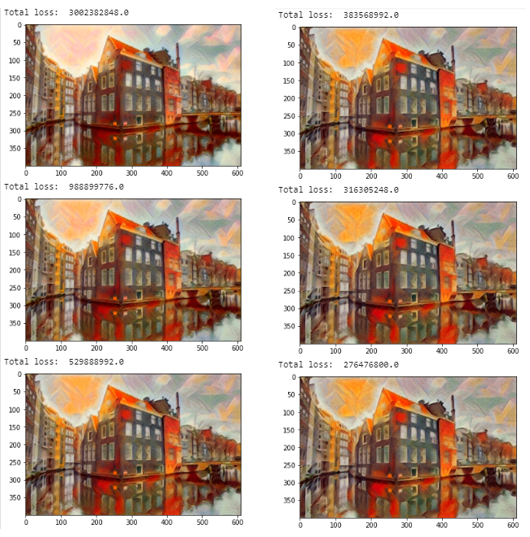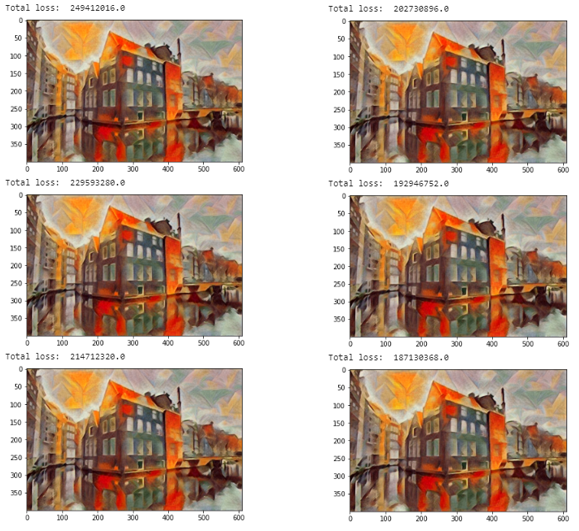## Conclusion

Great work! The loss is reducing after every epoch. Play with your creation by adjusting weights and learning rate. You may go for an LBFGS optimizer.

This guide gave you a general idea of how to code NST using PyTorch. I would recommend going through the NST using TensorFlow for a better understanding of the terms involved (losses, VGG-Net, cost function, etc.).

I hope you learned something new today. If you need any help with machine learning implementations, feel free to contact me.